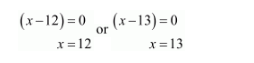# In a class test, the sum of Shefali's marks in Mathematics and English is 30.

Question:

In a class test, the sum of Shefali's marks in Mathematics and English is 30. Had she got 2 marks more in mathematics and 3 marks less in English, the product of her marks would have been 210. Find her marks in two subjects.

Solution:

Let marks obtained by Shefali in mathematics be $x$, then in english $=(30-x)$

It is given that,

$(x+2) \times(30-x-3)=210$

$(x+2) \times(27-x)=210$

$27 x-x^{2}+54-2 x=210$

$-x^{2}+25 x+54-210=0$

$-\left(x^{2}-25 x+156\right)=0$

$x^{2}-25 x+156=0$

$x^{2}-12 x-13 x+156=0$

$x(x-12)-13(x-12)=0$

$x(x-12)-13(x-12)=0$

$(x-12)(x-13)=0$Therefore, when $x=12$ then

$(30-x)=(30-12)$

$=18$

Hence, marks in mathematics $x=12$ and marks in science $=18$.

Or,

when $x=13$ then

$(30-x)=(28-13)$

$=17$

Hence, marks in mathematics $x=13$ and marks in science $=17$.

$(x-12)(x-13)=0$